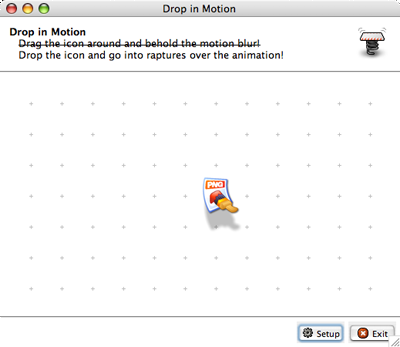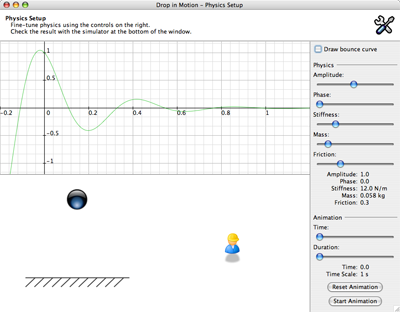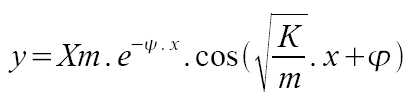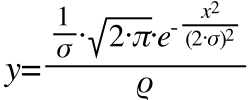## Real World Physics in Swing (Demo)

You can run the WebStart demo or, as usual, download its source code.

More and more applications offer complex interactions between the user and the GUI. Unfortunately, most of these interactions provide poor visual feedback. Some software companies are already ahead of most of us and provide interesting effects. Take for instance Apple and its animated drag and drop : when you drop something in a place it doesn't belong to you can see the item go back to its original position, effectively stressing the difference between a failed drag and a drop and a successful one. Microsoft Max also provide innovative visual feedback. When you display one of your photo albums, you can change the zoom level. As applications of the same kind, Max reorganizes the gallery according to the zoom level. Only it is animated and it is not annoying as some Windows XP effects can be.

So why couldn't we look at the “real” world and try to reproduce its behaviors? The Drop In Motion demo is an example of what we can do. The basic idea is that when you drag and drop something, it should behave like a real object. Therefore, when you initiate a drag gesture, you literally take the object otherwise you would rub it. Then, when you move the object around you should see a motion blur. Finally, when you drop it, it should fall and even bounce. This demo implements two of these behaviors. I have some code ready for the motion blur but I didn't take the time to enable it in the final demo, hence the stroked text in the first window. Take a look at the source code and you should find a runnable class showing two different implementation of the motion blur. I'll post a new version of the demo with motion blur enabled as soon as possible.

In the meantime you can still play with the physics law I implemented. The first screen you see when you start the demo is the following:Click on the icon, drag it around and drop it to witness a nice animation of the icon bouncing on the window. Before you get tired of tossing the icon, click on the Setup button to bring another window:This window lets you tune the equation used to render the animation. I used the physics law of a damping oscillator, that is a spring. Take a look at the bottom of the window: the dark sphere is animated, when you move the appropriate slider or hit the buttons, as if it was tied to a spring. You can change the different settings and go back to the first window to see how it affects the animation. You should know that it will work best when your values draw a graph where `0 <= y <= 1` for `0 <= x <= 1` (or `f([0, 1])->[0, 1]`). Pan and zoom the graph with the left mouse click and the mouse wheel to adjust the values precisely. Please note that the green graph is exactly the one used by the animation. It uses in fact `abs(f(x))` which you can see by checking the Draw bounce curve box. The most interesting parameters are the stiffness of the spring, the mass of the object and the friction of the environment. Finally, the animation parameters do not affect the first window (even though the time scale, or duration of the animation, should).

I used the equation of a spring because it was the only one I could easily remember and giving the appropriate graph:Parameters of this equation are:

• Xm: amplitude
• ψ: friction
• K: spring's stiffness
• m: mass of the object
• φ: phase

That said, the code can use any equation. You could for instance substitute our damping oscillator by a linear equation like `f(x)=x` or the one I used to render the CD Shelf:The demo classes take an AbstractEquation as a parameter. An AbstractEquation is just the implementation of the interface Equation along with a few methods to handle listeners:

```public interface Equation {
public double compute(double variable);
}
```

Here is the complete source code of the damping oscillator:

```public class DampingOscillatorEquation extends AbstractEquation {
public static final String PROPERTY_AMPLITUDE = "amplitude";
public static final String PROPERTY_PHASE = "phase";
public static final String PROPERTY_STIFFNESS = "stiffness";
public static final String PROPERTY_MASS = "mass";
public static final String PROPERTY_FRICTION_MULTIPLIER = "friction_multiplier";

// exposed parameters
private double amplitude;
private double phase;
private double stiffness;
private double mass;
private double frictionMultiplier;

// internal parameters
private double pulsation;
private double friction;

public DampingOscillatorEquation(double amplitude, double frictionMultiplier,
double mass, double rigidity, double phase) {
this.amplitude = amplitude;
this.frictionMultiplier = frictionMultiplier;
this.mass = mass;
this.phase = phase;
this.stiffness = rigidity;

computeInternalParameters();
}

private void computeInternalParameters() {
// never call computeFriction() without
// updating the pulsation
computePulsation();
computeFriction();
}

private void computePulsation() {
this.pulsation = Math.sqrt(stiffness / mass);
}

private void computeFriction() {
this.friction = frictionMultiplier * pulsation;
}

public double compute(double x) {
double y = amplitude * Math.exp(-friction * x) *
Math.cos(pulsation * x + phase);
return y;
}

public double getAmplitude() {
return amplitude;
}

public void setAmplitude(double amplitude) {
double oldValue = this.amplitude;
this.amplitude = amplitude;
firePropertyChange(PROPERTY_AMPLITUDE, oldValue, amplitude);
}

public double getFrictionMultiplier() {
return frictionMultiplier;
}

public void setFrictionMultiplier(double frictionMultiplier) {
double oldValue = this.frictionMultiplier;
this.frictionMultiplier = frictionMultiplier;
computeInternalParameters();
firePropertyChange(PROPERTY_FRICTION_MULTIPLIER,
oldValue, frictionMultiplier);
}

public double getMass() {
return mass;
}

public void setMass(double mass) {
double oldValue = this.mass;
this.mass = mass;
computeInternalParameters();
firePropertyChange(PROPERTY_MASS, oldValue, mass);
}

public double getPhase() {
return phase;
}

public void setPhase(double phase) {
double oldValue = this.phase;
this.phase = phase;
firePropertyChange(PROPERTY_PHASE, oldValue, phase);
}

public double getStiffness() {
return stiffness;
}

public void setStiffness(double rigidity) {
double oldValue = this.stiffness;
this.stiffness = rigidity;
computeInternalParameters();
firePropertyChange(PROPERTY_STIFFNESS, oldValue, rigidity);
}
}
```

The single constructor accepts the values you can read in the setup window. Here is the default oscillator used in the demo:

```private void setupEquations() {
damping = new DampingOscillatorEquation(1.0, 0.3, 0.058, 12.0, 0.0);
bouncer = new BouncerEquation(1.0, 0.3, 0.058, 12.0, 0.0);
}
```

You can use the setup window to find values you like and replace mine. You can also easily adapt the simulator to use other equations. It would be best if AbstractEquation provided a way to register parameters with a name and a description so that the UI could be automatically generated from the equation itself. Anyway, once your equation is set up you must call compute() to animate your object:

```private void drawItem(Graphics2D g2) {
double position = equation.compute(time * timeScale);
// ...
}
```

The variable time is a double value between 0.0 and 1.0 whereas timeScale is a time multiplier to change the duration of the animation. Its value is 1.0 by default and you can change it in the first window by changing the code of PlaygroundPanel, which inherits from AbstractSimulator, as both animated pictures in the setup window. The resulting value, which I consider comprised between 0.0 and 1.0, is used as a modifier for two properties of the icon, its size and the distance of the shadow. When looking at the animation, you would expect the icon to be enlarged when you pick it up. Actually doing so is not the best solution. Instead, the icon is shrunk when it lies on the “ground” and it gets its original size when you pick it up. Reducing a picture gives a better visual result than enlarging it.

Stay tuned for the motion blur, and read the code of this demo :)